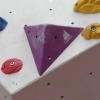# Resources tagged with: Vector algebra

Filter by: Content type:
Age range:
Challenge level:

There are 7 NRICH Mathematical resources connected to Vector algebra, you may find related items under Vectors and Matrices.

Broad Topics > Vectors and Matrices > Vector algebra### Vector Journeys

##### Age 14 to 18Challenge Level

Charlie likes to go for walks around a square park, while Alison likes to cut across diagonally. Can you find relationships between the vectors they walk along?### Tetra Perp

##### Age 16 to 18Challenge Level

Show that the edges AD and BC of a tetrahedron ABCD are mutually perpendicular when: AB²+CD² = AC²+BD².### Three by One

##### Age 16 to 18Challenge Level

There are many different methods to solve this geometrical problem - how many can you find?### Flexi Quads

##### Age 16 to 18Challenge Level

A quadrilateral changes shape with the edge lengths constant. Show the scalar product of the diagonals is constant. If the diagonals are perpendicular in one position are they always perpendicular?### Quaternions and Reflections

##### Age 16 to 18Challenge Level

See how 4 dimensional quaternions involve vectors in 3-space and how the quaternion function F(v) = nvn gives a simple algebraic method of working with reflections in planes in 3-space.### Quaternions and Rotations

##### Age 16 to 18Challenge Level

Find out how the quaternion function G(v) = qvq^-1 gives a simple algebraic method for working with rotations in 3-space.### Flexi Quad Tan

##### Age 16 to 18Challenge Level

As a quadrilateral Q is deformed (keeping the edge lengths constnt) the diagonals and the angle X between them change. Prove that the area of Q is proportional to tanX.